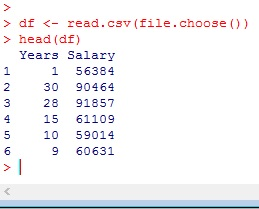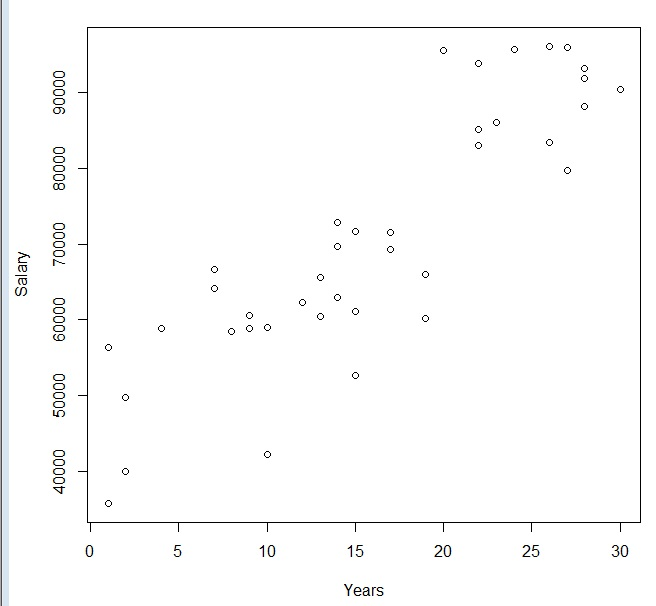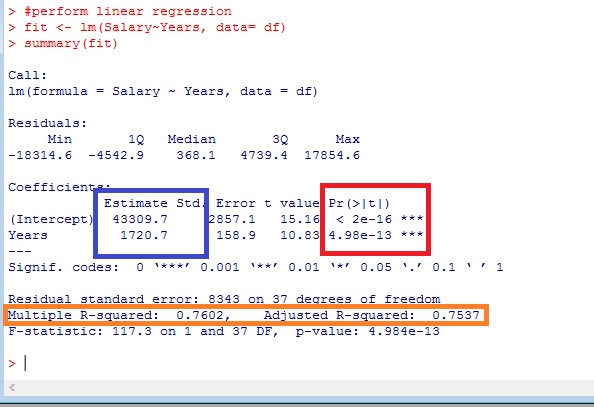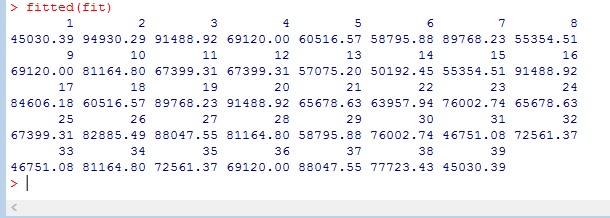# R: Simple Linear Regression

Linear Regression is a very popular prediction method and most likely the first predictive algorithm most be people learn. To put it simply, in linear regression you try to place a line of best fit through a data set and then use that line to predict new data points.If you are new to linear regression or are in need of a refresher, check out my lesson on Linear Regression in Excel where I go much deeper into the mechanics: Linear Regression using Excel

## Get the Data

Let’s upload our file into R

```df <- read.csv(file.choose())Now our data file contains a listing of Years a person has worked for company A and their Salary.

### Check for linear relationship

With a 2 variable data set, often it is quickest just to graph the data to check for a possible linear relationship.

```#plot data
attach(df)
plot(Years, Salary)```

Looking at the plot, there definitely appears to be a linear relationship. I can easily see where I could draw a line through the data.An even better way to do it is to check for correlation. Remember the closer to 1, the better the correlation found in the data.

```#check for correlation
cor(Years, Salary)```Since our correlation is so high, I think it is a good idea to perform an linear regression.

## Linear Regression in R

A linear regression in R is pretty simple. The syntax is lm(y, x, data)

```#perform linear regression
fit <- lm(Salary~Years, data= df)
summary(fit)```Now let’s take a second to break down the output.

The red box shows my P values. I want to make sure they are under my threshold (usually 0.05). This becomes more important in multiple regression.

The orange box shows my R-squared values. Since this is a simple regression, both of these numbers are pretty much the same, and it really doesn’t matter which one you look at. What these numbers tell me is how accurate my prediction line is. A good way to look at them for a beginner is to consider them to be like percentages. So in our example, our prediction model is 75-76% percent accurate.

Finally, the blue box are your coefficients. You can use these numbers to create your predictive model. Remember the linear equation: Y = mX + b? Well using your coefficients here our equation now reads Y = 1720.7X + 43309.7

## Predictions

You can use fitted() to show you how your model would predict your existing data

`fitted(fit)`You can also use the predict command to try a new value

`predict(fit, newdata =data.frame(Years= 40))`## Let’s graph our regression now.

```plot(Years, Salary)

abline(fit, col = 'red')```## The Residuals Plot

I am not going to go too deep into the weeds here, but I want to show you something cool.

```layout(matrix(c(1,2,3,4),2,2))  # c(1,2,3,4) gives us 4 graphs on the page,

#2,2 - graphs are 2x2
plot(fit)```

I promise to go more into this in a later lesson, but for now, I just want you to note the numbers you see popping up inside the graphs. (38,18,9) – These represent outliers. One of the biggest problems with any linear system is they are easily thrown off by outliers. So you need to know where you outliers are.If you look at the points listed in your graphs in your data, you will see why these are outliers. Now while this doesn’t tell you what to do about your outliers, that decision has to come from you, it is a great way of finding them quickly.## The Code

```# upload file

#plot data
attach(df)
plot(Years, Salary)

#check for correlation
cor(Years, Salary)

#perform linear regression
fit <- lm(Salary~Years, data= df)
summary(fit)

#see predictions
fitted(fit)

predict(fit, newdata =data.frame(Years= 40))

#plot regression line
plot(Years, Salary)

abline(fit, col = 'red')

layout(matrix(c(1,2,3,4),2,2))
plot(fit)

df```

## 3 thoughts on “R: Simple Linear Regression”

1.Vishal

could you please post an article on outlier treatment for a regression problem in R?

1.Anonymous

pbhaa

2.Anonymous

nenjinakath lalettan